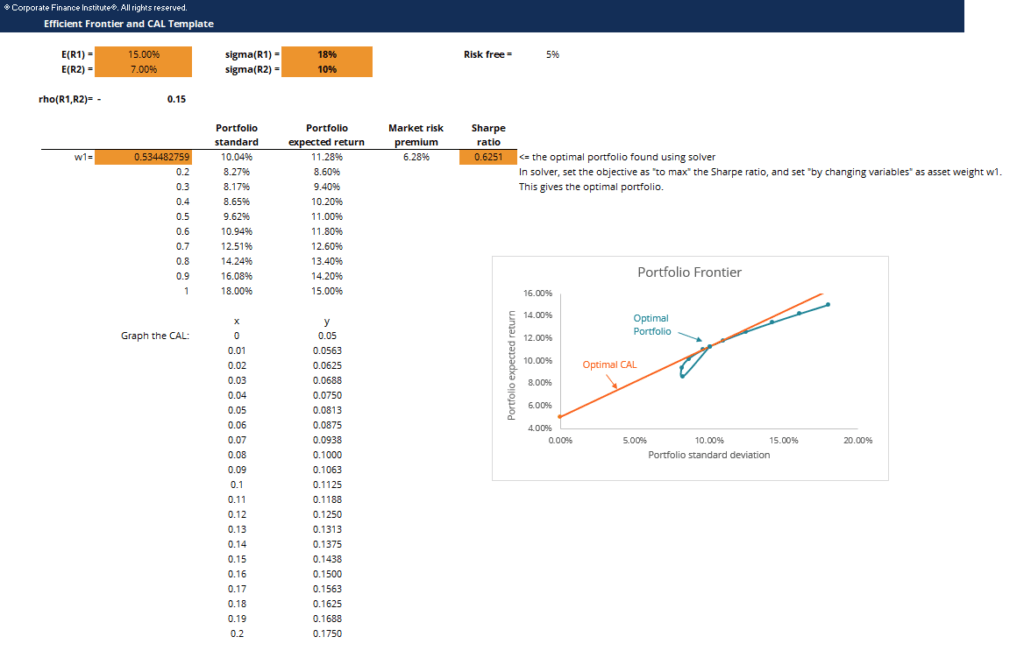# Efficient Frontier and CAL Template

## Efficient Frontier and CAL Template

This efficient frontier and CAL template demonstrates how to construct a portfolio frontier and CAL line chart to find the optimal portfolio.

Below is a screenshot of the efficient frontier and CAL template:### The efficient frontier

A portfolio frontier is a graph that maps out all possible portfolios with different asset weight combinations, with levels of portfolio standard deviation graphed on the x-axis and portfolio expected return on the y-axis.

### Capital allocation line (CAL)

The Capital Allocation Line (CAL) is a line that graphically depicts the risk-and-reward profile of risky assets, and can be used to find the optimal portfolio. The formula of CAL line is: E(Rc) = Rf + Spσ(Rc)

The slope of the line, Sp, is called the Sharpe ratio, or reward-to-risk ratio. The Sharpe ratio measures the increase in expected return per unit of additional standard deviation.

### Optimal portfolio

The optimal portfolio consists of a risk-free asset and an optimal risky asset portfolio. The optimal risky asset portfolio is at the point where the CAL is tangent to the efficient frontier. This portfolio is optimal because the slope of CAL is the highest, which means we achieve the highest returns per additional unit of risk.

### More Free Templates

For more resources, check out our business templates library to download numerous free Excel modeling, PowerPoint presentation and Word document templates.

• Excel Modeling Templates
• PowerPoint Presentation Templates
• Transaction Document Templates

### Financial Analyst Certification

Become a certified Financial Modeling and Valuation Analyst (FMVA)® by completing CFI’s online financial modeling classes and training program!